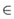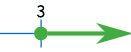Set-Builder Notation

How to describe a set by saying what properties its members have.A Set is a collection of things (usually numbers).

Example: {5, 7, 11} is a set.

But we can also "build" a set by describing what is in it.

Here is a simple example of set-builder notation:It says "the set of all x's, such that x is greater than 0".

In other words any value greater than 0

Notes:

• The "x" is just a place-holder, it could be anything, such as { q | q > 0 }
• Some people use ":" instead of "|", so they write { x : x > 0 }

Type of Number

It is also normal to show what type of number x is, like this:• Themeans "a member of" (or simply "in")
• Theis the special symbol for Real Numbers.

So it says:

"the set of all x's that are a member of the Real Numbers,
such that x is greater than or equal to 3"

In other words "all Real Numbers from 3 upwards"

There are other ways we could have shown that:

On the Number Line it looks like:In Interval notation it looks like: [3, +∞)

Number Types

We saw(the special symbol for Real Numbers). Here are the common number types:Natural Numbers Integers Rational Numbers Real Numbers Imaginary Numbers Complex Numbers

Example: { k| k > 5 }

"the set of all k's that are a member of the Integers, such that k is greater than 5"

In other words all integers greater than 5.

This could also be written {6, 7, 8, ... } , so:

{ k| k > 5 } = {6, 7, 8, ... }

Why Use It?

When we have a simple set like the integers from 2 to 6 we can write:

{2, 3, 4, 5, 6}

But how do we list the Real Numbers in the same interval?

{2, 2.1, 2.01, 2.001, 2.0001, ... ???

So instead we say how to build the list:

{ x| x ≥ 2 and x ≤ 6 }
Start with all Real Numbers, then limit them between 2 and 6 inclusive.

We can also use set builder notation to do other things, like this:

{ x| x = x2 } = {0, 1}
All Real Numbers such that x = x2
0 and 1 are the only cases where x = x2

Another Example:

Example: x ≤ 2 or x > 3

Set-Builder Notation looks like this:

{ x| x ≤ 2 or x >3 }

On the Number Line it looks like:Using Interval notation it looks like:

(−∞, 2]  U  (3, +∞)

We used a "U" to mean Union (the joining together of two sets).

Defining a Domain

Set Builder Notation is very useful for defining domains.In its simplest form the domain is the set of all the values that go into a function.

The function must work for all values we give it, so it is up to us to make sure we get the domain correct!

Example: The domain of 1/x

1/x is undefined at x=0 (because 1/0 is dividing by zero).

So we must exclude x=0 from the Domain:

The Domain of 1/x is all the Real Numbers, except 0

We can write this as

Dom(1/x) = {x| x ≠ 0}

Example: The domain of g(x)=1/(x−1)

1/(x−1) is undefined at x=1, so we must exclude x=1 from the Domain:

The Domain of 1/(x−1) is all the Real Numbers, except 1

Using set-builder notation it is written:

Dom( g(x) ) = { x| x ≠ 1}

Example: The domain of √x

Is all the Real Numbers from 0 onwards, because we can't take the square root of a negative number (unless we use Imaginary Numbers, which we aren't).

We can write this as

Dom(√x) = {x| x ≥ 0}

Example The domain of f(x) = x/(x2 − 1)

To avoid dividing by zero we need: x2 - 1 ≠ 0

Factor: x2 - 1 = (x−1)(x+1)

(x−1)(x+1) = 0 when x = 1 or x = −1, which we want to avoid!

So:

Dom( f(x) ) = {x| x ≠ 1, x ≠ −1}